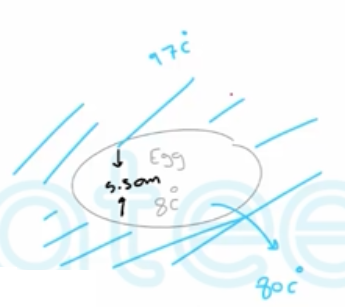Need Help?

Subscribe to Thermodynamics

###### \${selected_topic_name}
• Notes

$\text { A I-kg block of iron is heated from } 25 \text { to } 75^{\circ} \mathrm{C} \text { . What is the change in the iron's total internal energy and enthalpy? }$

$A-3 b \longrightarrow c=0.45 \mathrm{kJ} / \mathrm{kg} . \mathrm{k}$

$\Delta H=\Delta U$$\longrightarrow \text { Solids }$

$∴ \quad \Delta H=\Delta V=m c \Delta T$

$=1 * 0.45 *(75-25)$

$=\quad 22.5 \mathrm{kJ}$

$\begin{array}{l}{\text { An ordinary egg can be approximated as a } 5.5 \text { -cm- diameter sphere. }} \\ {\text { The egg is initially at a uniform temperature of } 8^{\circ} \mathrm{C} \text { and is dropped into boiling water }} \\ {\text { at } 97^{\circ} \mathrm{C} \text { . Taking the properties of the egg to be } \mathrm{p}=1020 \mathrm{kg} / \mathrm{m} 3 \text { and } \mathrm{cp}=3.32 \mathrm{kJ} / \mathrm{kg}-\mathrm{C} \text { , }}\end{array}$

$\begin{array}{l}{\text { determine how much heat is transferred to the egg by the time }} \\ {\text { the average temperature of the egg rises to } 80^{\circ} \mathrm{C} \text { . }}\end{array}$$E_{i n-} E_{o u t}= \Delta E_{system}$

$Q_{\text { in }}=\Delta U_{\text { egg }}=m\left(u_{2}-u\right)$

$=m\left(T_{2}-T_{1}\right)$

$Q_{i n}=m c p\left(T_{2}-T_{1}\right)$

$m=\rho v=\rho+\frac{\pi D^{3}}{6}$$=(1020) \frac{\pi(0.055)^{3}}{6}=0.0889 \mathrm{kg}$

$Q_{i n}=0.0889 * 3.32(80-8)=21.2 kJ$